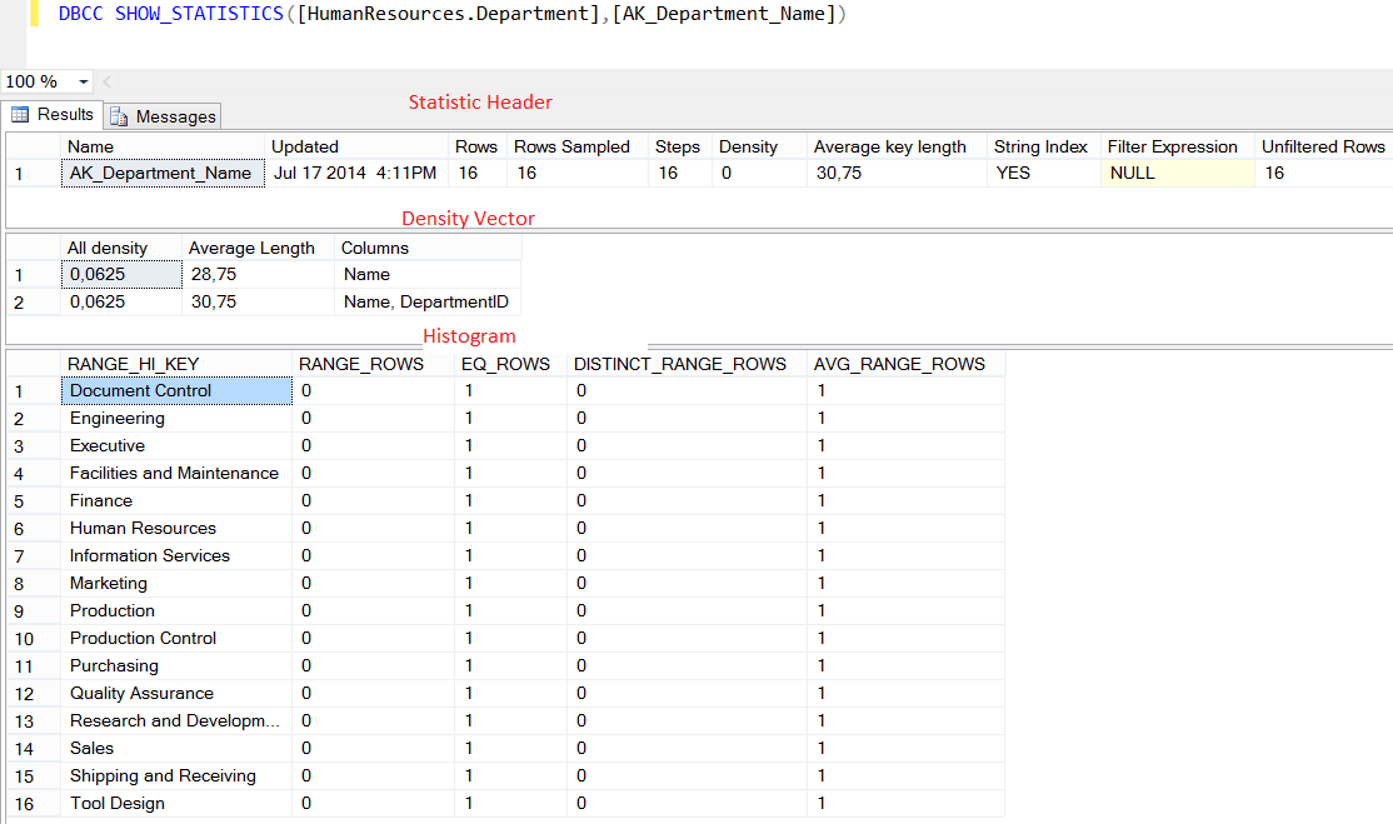Thursday , September 29 2022

# DBCC SHOW_STATISTICS in SQL Server

With DBCC SHOW_STATISTICS we can get detailed information about a statistic. As many of you know, statistics have one of the most important roles in the decision making of the sql server engine when creating a query plan. The SQL Server Engine looks at the statistics and decides whether or not an index should be used. So first of all, our statistics need to be up to date.

Before you read this article, I recommend that you read the following articles to better understand why you need this command.

DBCC SHOW_STATISTICS shows detailed information about a statistic in a table or indexed view.

The output of this command consists of 3 elements.

• Density Vector
• Histogram

Let us explain the above concepts and details through an example. Run the following command in the AdventureWorks database.

In this command,

HumanReources is the schema name,

Department is the table name and

AK_Department_Name is the statistics name.

We see a result set as follows.In the following tables, you can see the descriptions of the columns in the result set.

 Statistic Header Name Statistic Name Updated Last update date of statistic Rows Shows the number of rows on the table or indexed view when the statistic were last updated. Rows Sampled The number of rows sampled when creating or updating statistics. You can find more information in the article “UPDATE STATISTICS in SQL Server” Step Specifies the number of steps in the histogram. We will mention later. Density Not used after SQL Server 2008. Average Key Length The average size of the columns of statistic(in bytes) String Index If the columns of the statistic are char, varchar, nchar, nvarchar, varchar (max), nvarchar (max), text, or ntext, the value of this column is YES. Filter Expression If the statistic is filtered, it shows the filter expression. Unfiltered Rows Shows the number of unfiltered rows. If there is no filter, it will show the number of rows in the corresponding table or indexed view.
 Density Vector All Density This refers to the value “1 / n”. “n” is the number of different records in the table. The closer this row is to 0, the more singularity is achieved. Average Length The average size of the columns of statistic(in bytes) Columns This column shows the corresponding columns.
 Histogram This is a graph showing the data distribution. When SQL Server generates a statistic, it first sorts the column values. It then divides the data into specific groups and sets the upper limit of each group. For example, consider a column with values ​​from 1 to 1000. In this column, statistics were grouped and divided into 100 group(1 to 10, 10 to 20…990 to 1000).  The first group(1 to 10) is defined as step 1. Because of we divide the statistic into 100 group, this means statistic has 100 steps. RANGE_HI_KEY This is the key value that specifies the upper limit for the Histogram step. So you can think of it as a line separating each step. RANGE ROWS Estimated number of rows per histogram step EQ_ROWS Number of records equal to each other at step limit DISTINCT_RANGE_ROWS Estimated number of non-duplicated rows per histogram step AVG_RANGE_ROWS Number of duplicated records in the corresponding step##### Author: dbtut

We are a team with over 10 years of database management and BI experience. Our Expertises: Oracle, SQL Server, PostgreSQL, MySQL, MongoDB, Elasticsearch, Kibana, Grafana.